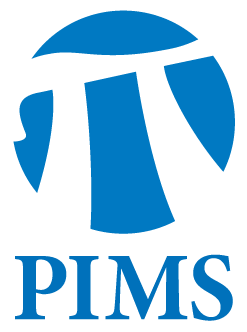## Discrete Math Seminar: Ryuhei Uehara

• Date: 06/19/2012
• Time: 14:30
Lecturer(s):
Ryuhei Uehara
Location:

Simon Fraser University

Topic:

The Graph Isomorphism Problem on Geometric graphs

Description:

Abstract:

The graph isomorphism (GI) problem asks if two given graphs are isomorphic or not. That is, it asks there exists a one-to-one mapping between two given graphs. The GI problem is quite basic, but it's time complexity isa long standing open problem. The problem is clearly in NP, but it is notknown to be NP-complete or not. The GI problem is one of candidates between the classes NP and P. The problem has four aspects from the viewpoints oftheoretical computer science. (1) What the computational complexity class that captures the GI problem? (2) How fast can we solve the problem (in exponential time)? (3) What the graph classes such that the GI problem is still as hard as general case? (4) What the graph classes such that the GI problem can be solved in polynomial time. In this talk, I focus on (3) and (4). If we can clarify this gap, it indicates the essential difficulty of the GI problem. Last few decades, many graph classes are proposed and investigated. I introduce some graph classes that have geometric characterization. Typical examples are interval graphs, that are intersection graphs of intervals, and chordal graphs, that are intersection graphs of subtrees of a tree. The GI problem can be solved in linear time for interval graphs, while the GI problem for chordal graphs is as hard as general case. I introduce basic techniques to show these results and some graph classes such thatthe complexity of the GI problem are not known.

Other Information: